# bbricks-getting-started

bbricks provides a collection of frequently used Bayesian parametric and nonparametric model structures, as well as a set of tools for common analytical tasks.

• Structures include linear Gaussian systems, Gaussian and Gaussian conjugate structure, Gaussian and Inverse-Wishart conjugate structure, Gaussian and Normal-Inverse-Wishart conjugate structure, Gaussian and Normal-Inverse-Gamma conjugate structure, Categorical and Dirichlet conjugate structure, Dirichlet Process on positive integers, Dirichlet Process in general, Hierarchical Dirichlet Process …
• Tasks include updating posteriors, calculating marginal likelihood, calculating posterior predictive densities, sampling from posterior distribution, sampling from posterior predictive distribution, calculating MAP estimates …

See Mindset for the idea behind bbricks and Examples to get started.

Note: This is a package designed for statisticians, and the ones who want to learn the basic Bayesian mindsets.

—-Installation—-

# install from CRAN:
install.packages("bbricks")
# or install development version from GitHub:
# install.packages("devtools")
devtools::install_github("chenhaotian/Bayesian-Bricks")

## Mindset

The idea of bbricks came from the fact that modeling in Bayesian statistics is nothing more than applying a set of tasks on a specific model structure.

Where the most frequently appeared tasks are:

• Update prior info into posterior when new samples are observed.
• Sample from the posterior distribution.
• Calculate marginal likelihood of the data set.
• Calculate posterior predictive densities from the posterior distribution.

And the model structures are always constituted by $$3$$ basic Bayesian modeling structures: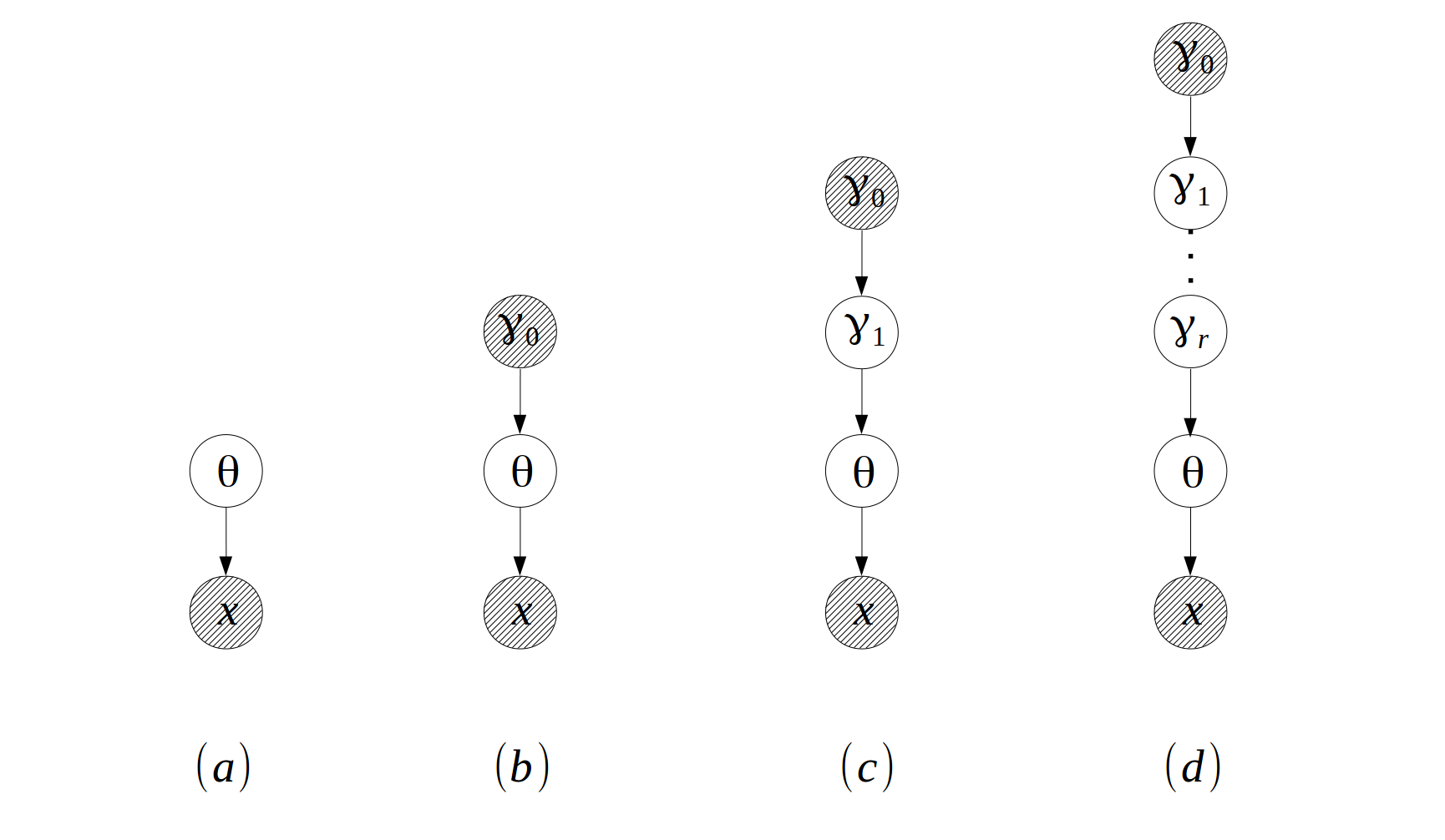Where

• $$(a)$$ is the most basic “parameter-observation” structure. Models like Gaussian, Gamma and Exponential are in this category.
• $$(b)$$ is the “prior-posterior” structure. Models like Gaussian-NIW(Gaussian observations with NIW prior), Categorical-Dirichlet(Categorical observations with Dirichlet prior) are in this category.
• $$(c)$$ is the “hierarchical-Bayesian” structure, and $$(d)$$ is the same hierarchical structure but with more hierarchies. Models like Hierarchical Dirichlet Process(HDP) and HDP with additional hidden layers are in this category.

bbricks tries to provide a type/class for each basic model structure and a function/method for each task.

See Examples for details.

## Examples

### Hierarchical Bayesian Linear Regression

This is an example from Hoff(2009). Where we want to examine the relationship between math score and another variable, socioeconomic status (SES) of students from $$J=100$$ different schools. The Conditional Probability Distributions (CPDs) of the model are defined as: \begin{align} x | \beta_j , \sigma^2,x & \sim Gaussian(X\beta_j,\sigma^2)\text{, }j = 1:J \\ \beta_j & \sim Gaussian(\mu,\Sigma)\text{, } j = 1:J\\ \sigma^2 & \sim InvWishart(v_s,S_s) \\ \mu,\Sigma & \sim NIW(m,k,v,S) \end{align}

Where $$x$$ is the math score, $$X$$ is a length $$2$$ row vector $$X = (1,\text{SES})^T$$ corresponding to each math score observation in $$x$$, NIW is Normal-Inverse-Wishart distribution, it’s density function is defined as: $p_{NIW}(\mu,\Sigma | m,k,v,S) = p_{Gaussian}(\mu|m,\Sigma/k)p_{InverseWishart}(\Sigma|v,S)$

The graphical model structure for this model is: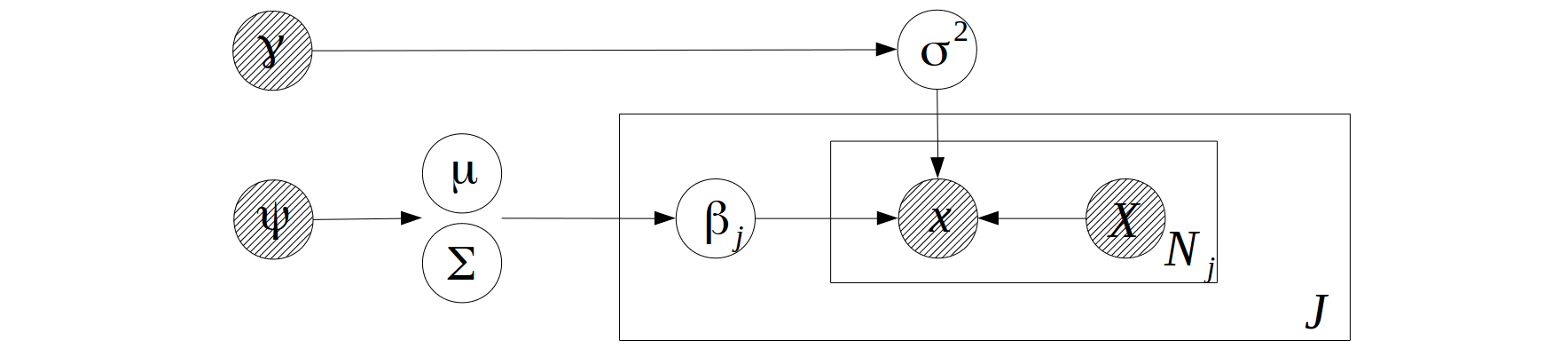Where $$\gamma = (v_s,S_s)$$, $$\Psi = (m,k,v,S)$$.

To enable sampling from this model, we first look at the CPDs of the random variables and their Markov blankets:

1. The Markov blanket for $$\beta_j,j=1:J$$ is $$x, X, \mu,\Sigma,\sigma^2$$, the corresponding CPD of the blanket is a linear Gaussian system. bbricks provides an object of type "LinearGaussianGaussian" (see ?LinearGaussianGaussian in R for details) to encode such a structure.
2. The Markov blanket for $$\mu,\Sigma$$ is $$\beta_{1:J},m,k,v,S$$, the corresponding CPDs of the blanket forms an Gaussian-NIW conjugate structure. bbricks provides an object of type "GaussianNIW" (see ?GaussianNIW in R for details) to encode such a structure.
3. The Markov blanket for $$\sigma^2$$ is $$\beta_{1:J},x,X,v_v,S_v$$, the corresponding CPDs of the blanket forms an Gaussian-InvWishart conjugate structure. bbricks provides an object of type "GaussianInvWishart" (see ?GaussianInvWishart in R for details) to encode such a structure.

Note that "LinearGaussianGaussian", "GaussianNIW" and "GaussianInvWishart" are all basic prior-posterior structure as shown in Mindset graph $$(b)$$. In bbricks, all objects representing structures same as graph $$(b)$$ are also of type "BasicBayesian". For example a "LinearGaussianGaussian" object is also an "BasicBayesian" object.

To estimate the posterior parameters $$(v_s,S_s,m,k,v,S)$$, the Gibbs sampling procedure goes as:

1. Sample $$\beta_j,j=1:J$$ from a LinearGaussianGaussian object which encodes the distribution of $$\beta_j | x, X, \mu,\Sigma,\sigma^2$$.
2. Sample $$\mu,\Sigma$$ from a GaussianNIW object which encodes the distribution of $$\mu,\Sigma | \beta_{1:J},m,k,v,S$$
3. Sample $$\sigma^2$$ from a GaussianInvWishart object which encodes the distribution of $$\sigma^2 | \beta_{1:J},x,X,v_v,S_v$$

R code:

## Gibbs sampling for hierarchical linear regression

library(bbricks)
## load some hierarchical linear regression data
## hlrData is a list of 3 numeric vectors
## see ?hlrData for details
data(hlrData)
x <- hlrData$mathScore #math score as the dependent variable X <- cbind(1,hlrData$socioeconomicStatus) #socioeconomic status as the independt variable
js <- hlrData$schoolID #school ID as the group IDs. J <- max(js) ## Initialization---------------------------------------------- ## initialize the Markov blanket of mu and Sigma ## the prior parameters are: m=0, k=0.0001, v=3, S=diag(1) objmS <- GaussianNIW(gamma = list(m =c(mean(hlrData$mathScore),0),k=0.0001,v=3,S=diag(2)))
## initialize the Markov blanket of sigma^2
## the prior parameters are: vs=2, Ss=diag(1)
objs <- GaussianInvWishart(gamma = list(mu=0,v=2,S=diag(1)))
## initialize mu and Sigma by sampling from the prior
muSigma <- rPosterior(objmS)
## initialize sigma^2 by sampling from the prior
sigma2 <- rPosterior(objs)
betaJ <- matrix(0,J,2)                  #place-holder the beta_j, j=1:J
epsilon <- x                            #place-holder for the random noises
## Main Gibbs loop---------------------------------------------
maxit <- 100                           #number of sampling iterations
burnin <- 50                           #number of burn-in samples
meanBeta <- betaJ                      #place-hoder for the sample means of beta
it <- 1
pb <- txtProgressBar(min = 0,max = maxit,style = 3)
while(it<=maxit){
## Step1: sample beta_j, j in 1:100
for(j in 1L:J){
objb <- LinearGaussianGaussian(gamma=list(Sigma=sigma2,m=muSigma$mu,S=muSigma$Sigma))
idx <- js == j
ss <- sufficientStatistics(obj = objb,x=x[idx],A=X[idx,,drop=FALSE])
posterior(obj = objb,ss = ss)
betaJ[j,] <- rPosterior(objb)
}
## calculate the sample mean
if(it>burnin) meanBeta <- meanBeta+betaJ/(maxit-burnin)
## Step2: sample mu and Sigma
ssmS <- sufficientStatistics(obj = objmS,x=betaJ)
posterior(obj = objmS,ss = ssmS)
muSigma <- rPosterior(obj = objmS)
## Step3: sample sigma^2
for(j in 1L:J){
idx <- js == j
epsilon[idx] <- x[idx]-X[idx,,drop=FALSE]%*%betaJ[j,]
}
sss <- sufficientStatistics(obj = objs,x=epsilon)
posterior(obj = objs,ss = sss)
sigma2 <- rPosterior(objs)
## increase iteration counter
it <- it+1
setTxtProgressBar(pb,it)
## if continue sampling, then discard the information in objmS and objs
## to make room for the new information in the next iteration.
if(it < maxit){
}
}
## plot the result
## gray lines are the betas of each school
## black line is the beta for all the data as a whole
plot(x=0, xlim = range(0.2,0.8),ylim = c(20,35),xlab = "socioeconomic status",ylab = "math score")
for(j in 1L:J)
abline(a=betaJ[j,2],b=betaJ[j,1],col="gray")
allSchools <- lm(x~X-1)coefficients abline(a=allSchools,b=allSchools,lwd=3) ### Estimate Cancer Mortality Rates with Hierarchical Bayesian This is an example from Johson and Albert(2006), where we want to estimate the cancer mortality rates of multiple cities with hierarchical Bayesian method. The model’s graph structure is:Where $$x$$ is a categorical random sample that takes one of two values: “death” or “no death”. There are $$K$$ cities in total, $$\pi_k,k=1:k$$ are the mortality rates of the cities. The CPDs are: \begin{align} x|\pi_k & \sim Categorical(\pi_k) \\ \pi_k | \alpha & \sim Dirichlet(\alpha) \\ \alpha | \eta & \sim Exponential(\eta) \end{align} To enable sampling from this model, we first look at the CPDs of the random variables and their Markov blankets: 1. The Markov blanket for $$\pi_k,k=1:K$$ is $$\alpha, x$$, the corresponding CPD of the blanket is a Categorical-Dirichlet conjugate structure. bbricks provides an object of type "CatDirichlet" (see ?CatDirichlet in R for details) to encode such a structure. 2. The Markov blanket for $$\alpha$$ is $$\pi_{1:K},\eta$$, the corresponding CPDs of the blanket is not very common thus not provided in bbricks, instead we can use Metropolis-Hastings algorithm to sample from it. Note that "CatDirichlet" is a basic prior-posterior structure as shown in Mindset graph $$(b)$$. In bbricks, all objects representing structures same as graph $$(b)$$ are also of type "BasicBayesian". For example a "CatDirichlet" object is also an "BasicBayesian" object. To estimate $$\pi_k,k=1...K$$, we use the following Gibbs sampling procedure: 1. sample $$\pi_k,k=1...K$$ from a CatDirichlet object which encodes the distribution of $$\pi_k | \alpha,x$$. 2. sample $$\alpha$$ from the distribution of $$\alpha|\eta,\pi_{1:K}$$ with and independent Metropolis-Hastings algorithm. see ?MetropolisHastings for details. R code: ## Estimate cancer mortality rates using Gibbs sampling library(bbricks) ## see ?cancerData for details data(cancerData) ## Step1: Initialization---------------------------------------------- K <- length(cancerData) #then number of cities eta <- 1 #assume eta is known, eta=1 ## initialize alpha, PI, and sufficient statistics a <- rexp(2,rate = eta) #initialize alpha PI <- matrix(0,K,2L) #initialize pi cityPrior <- CatDirichlet(gamma = list(alpha=a,uniqueLabels=c("death","no death"))) citySS <- lapply(cancerData,function(x){sufficientStatistics(obj = cityPrior,x=x)}) #sufficient statistics of each city ## initialize functions used in Metropolis-Hastings, see ?MetropolisHastings for details ## density of the target distribution dp <- function(a){ if(any(a<0)) -Inf else sum(dDir(x=PI,alpha = a,LOG = TRUE))+sum(dexp(x=a,rate = eta,log = TRUE)) } ## density of the proposal distribution dq <- function(anew,a){1} #use a independent proposal ## random sample generator of the proposal distribution rq <- function(x){ c(runif(1,x-1,x+1), runif(1,x-1,x+1)) } ## Step2: main Gibbs sampling loop between alpha and pi -------------- maxit <- 1000 burnin <- 500 #number of burn-in samples meanPI <- numeric(K) #place-hoder for the sample mean it <- 1 while(it<=maxit){ ## Step1: sample pi from p(pi|a,x)------------- for(k in 1L:K){ posterior(obj = cityPrior,ss=citySS[[k]]) PI[k,] <- rDir(n=1,alpha = cityPriorgammaalpha) posteriorDiscard(obj = cityPrior,ss=citySS[[k]]) } ## calculate the sample mean if(it>burnin) meanPI <- meanPI+PI[,1]/(maxit-burnin) ## Step2: sample a from p(a|pi,g)-------------- ## use Metropolis-Hastings a <- MetropolisHastings(nsamples = 1,xini = a,dp=dp,dq=dq,rq=rq) ## increase iteration counter it <- it+1 } ## Step3: plot the result--------------------------------------------- ## black bars are the sample mean from the hierarchical Bayesian model ## blue bars are the MLE of the mortality rates. plot(1:K,meanPI,type = "h",xlab = "city",ylab = "mortality rate",lwd=3) lines(1:K+0.2,sapply(cancerData,function(l){sum(l=="death")/length(l)}),type = "h",col = "blue",lwd = 3) legend(1, 0.005, legend=c("Sample Mean", "MLE"),col=c("black", "blue"), lty=c(1,1), cex=1,lwd = 3) ### Mixture of Gaussian A mixture of Gaussian has the following graph structure: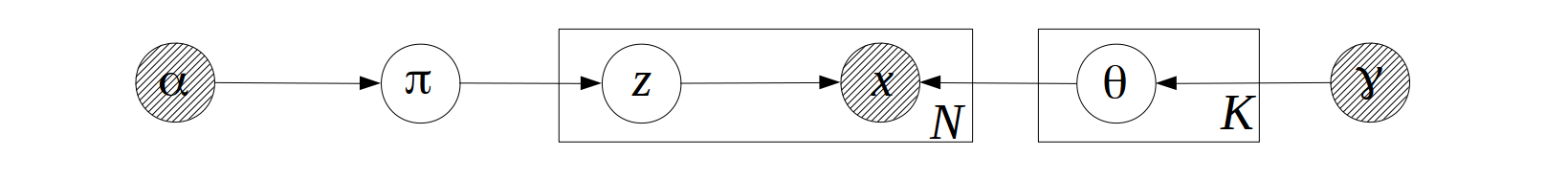Where there are $$K$$ Gaussian components/groups. $$x$$ is an Gaussian observation, $$z=1,...,K$$ is the hidden group label, $$\pi$$ is the component weights (or the group label distribution). $$\theta=(\mu,\Sigma)$$ are the observation distribution parameters. $$\alpha$$ and $$\gamma$$ are prior parameters. The CPDs are: \begin{align} \pi |\alpha &\sim Dirichlet(\alpha) \\ z|\pi & \sim Categorical(\pi) \\ \theta_z | \gamma &\sim NIW(\gamma) \\ x| z,\theta_z &\sim Gaussian(\theta_z) \end{align} Where $$NIW(\gamma)$$ is the Normal-Inverse-Wishart distribution with parameter $$\gamma = (m,k,v,S)$$. $$m$$ is a numeric vector representing the “location parameter”, $$S$$ is a symmetric positive definitive matrix representing the “scale parameter”, $$k$$ and $$v$$ are degree of freedoms. For a NIW sample $$\theta=(\mu,\Sigma)$$, it’s density function is defined as: $p_{NIW}(\mu,\Sigma | m,k,v,S) = p_{Gaussian}(\mu|m,\Sigma/k)p_{InverseWishart}(\Sigma|v,S)$ A mixture model can be see as a combination of two “prior-posterior” structures(As shown in Mindset graph $$(b)$$): One Categorical-Dirichlet structure $$\alpha \rightarrow \pi \rightarrow z$$ for the hidden cluster labels. and one Gaussian-NIW structure $$\gamma \rightarrow \theta \rightarrow x$$ for the observation distribution. In bbricks these two structures are initialized with a "CatDirichlet" object and a "GaussianNIW" object. To estimate $$\pi$$ and $$\theta$$, we use the following EM procedure: 1. E-step: calculate $$p(z|\theta,\pi,x)$$ and the expected sufficient statistics$$E_z(T(z))$$ and $$E_z(T(x))$$. 2. M-step: Based on the expected sufficient statistics to get an MAP estimate of $$\theta$$ and $$\pi$$ R code: ## Get the MAP estimate of pi and theta using EM algorithm. library(bbricks) ## load some mixture of Gaussian samples. ## mmData is a numeric matrix with 2 columns, each row is a sample ## see ?mmData for details data(mmData) K <- 4L #number of clusters(mixtures components) z <- matrix(runif(nrow(mmData)*K),nrow(mmData),K) #the expected cluster label of each observation allK <- 1L:K #temp variable, all component labels allZ <- rep(allK,each=nrow(mmData)) #temp variable, all possible cluster labels for all observations ## z, pi and alpha are distributed as a Categorical-Dirichlet sturcture: mc <- CatDirichlet(gamma = list(alpha=0.5,uniqueLabels=allK)) # create a CatDirichlet object to track the posterior info, see ?CatDirichlet for details ## each component distribution is a Gaussian-NIW structure: ec <- replicate(K,GaussianNIW(gamma = list(m=c(0,0),k=0.00001,v=2,S=diag(2)))) # create a GaussianNIW object to track the posterior info of each mixture component, see ?GaussianNIW for details mcMAP <- MAP(mc) #initialize the MAP estimate of pi ecMAP <- replicate(K,list(muMAP=runif(2),sigmaMAP=diag(2)),simplify = FALSE) #initialize the MAP estimate of theta ## The main EM loop maxit <- 100 #number of EM loops it <- 1 while(it<=maxit){ ## E-step--------------------------------------------------------- ## calculate the expected cluster labels: p(z|pi,theta) for(k in allK) z[,k] <- dGaussian(x=mmData,mu = ecMAP[[k]]muMAP,Sigma=ecMAP[[k]]sigmaMAP)+log(mcMAP[k]) z <- exp(z-logsumexp(z)) #use logsumexp() to avoid numerical underflow ## calculate the expected sufficient statistics ssComponents <- lapply(allK,function(k){ sufficientStatistics_Weighted(obj = ec[[k]],x=mmData,w=z[,k]) }) #the expected sufficient statistics of each Gaussian component ssPi <- sufficientStatistics_Weighted(obj = mc,x=allZ,w=as.vector(z)) #the expected sufficient statistics of the cluster label distribution ## M-step--------------------------------------------------------- ## use the sufficient statistics to update the prior distributions: for(k in allK) posterior(obj = ec[[k]],ss=ssComponents[[k]]) #update component distributions posterior(obj = mc,ss = ssPi) #update cluster label distribution ## calculate the MAP estimates from posterior: mcMAP <- MAP(mc) ecMAP <- lapply(ec,MAP) ## Reset the priors for next EM loop----------------------------------------- ## to prepare for the next EM iteration, discard the sufficient statistics info from the posteriors: for(k in allK) posteriorDiscard(obj = ec[[k]],ss=ssComponents[[k]]) posteriorDiscard(obj = mc,ss = ssPi) ## increase the iteration counter it <- it+1 } plot(mmData,col=apply(z,1,which.max)) #plot the best estimates mcMAP #the MAP estimate of pi ecMAP #the MAP estimate of theta_z #### Dirichlet Process Mixture Model The graph structure of Dirichlet Process Mixture Model(DP-MM) is exactly the same as a standard mixture model, except that the number of mixture components is not predetermined: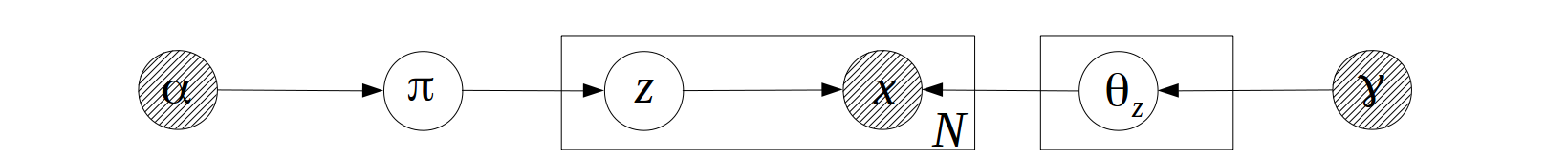The CPDs of a DP-MM is similar to the ones shown in Mixture of Gaussian, the only difference is the distribution of $$\pi|\alpha$$ is a Dirichlet process rather than a Dirichlet distribution, for example if the observations are Gaussian distributed, the CPDs will be: \begin{align} \pi |\alpha &\sim DP(\alpha) \\ z|\pi & \sim Categorical(\pi) \\ \theta_z | \gamma &\sim NIW(\gamma) \\ x| z,\theta_z &\sim Gaussian(\theta_z) \end{align} Where $$DP(\alpha)$$ is a Dirichlet process on positive integers with “concentration parameter” $$\alpha$$, the “base measure”, which is an uniform distribution on positive integers, is omitted from the formula. $$NIW(\gamma)$$ is the Normal-Inverse-Wishart distribution with parameter $$\gamma = (m,k,v,S)$$. $$m$$ is a numeric vector representing the “location parameter”, $$S$$ is a symmetric positive definitive matrix representing the “scale parameter”, $$k$$ and $$v$$ are degree of freedoms. A DP-MM can be see as a combination of two “prior-posterior” structures(As shown in Mindset graph $$(b)$$): One Categorical-DirichletProcess structure for the hidden cluster label distribution $$\alpha \rightarrow \pi \rightarrow z$$, which we call it a “DP on positive integers”. And one structure for the observation distribution $$\gamma \rightarrow \theta_z \rightarrow x$$. In bbricks, “DP on positive integers” is represented by an object of type "CatDP". To further simplify the calculations, bbricks also provides an "DP" type to represent all Dirichlet process structures. An object of type "DP" is in essence a combination of a "CatDP" object, which encodes the $$\alpha \rightarrow \pi \rightarrow z$$ structure, i.e. a Dirichlet process on positive integers, and an arbitrary "BasicBayesian" object, which encodes the $$\gamma \rightarrow \theta_z \rightarrow x$$ structure. (in bbricks, all models with same structure as Mindset graph $$(b)$$ are "BasicBayesian"s, such as "GaussianNIW", "GaussianNIG", "CatDirichlet" and even "CatDP") . To estimate $$z$$, we use the following collapse Gibbs sampling procedure: 1. sample $$z_i$$ from $$z_i|z_{-i},\alpha,x,\gamma$$. R code: ## Learn DP-MM posteriors using Gibbs sampling library(bbricks) ## load some mixture of Gaussian samples. ## mmData is a numeric matrix with 2 columns, each row is a sample ## see ?mmData for details data(mmData) maxit <- 100 #number of total samples burnin <- 50 #number of burnin samples ## Step1: Initialization ----------------------------------------- obj <- DP(gamma = list(alpha=10,H0aF="GaussianNIW",parH0=list(m=c(0,0),k=0.001,v=2,S=diag(2)))) #create a DP object to track all the changes, the DP object in this case is a combination of a CatDP object and a GaussianNIW object z <- matrix(1L,nrow(mmData),maxit-burnin) #place-holder for the sampled z ss <- sufficientStatistics(obj,x=mmData,foreach = TRUE) #sufficient statistics of each observed sample N <- nrow(mmData) for(i in 1L:N){ # initialize labels before Gibbs sampling z[i,1] <- rPosteriorPredictive(obj = obj,n=1,x=mmData[i,,drop=FALSE]) posterior(obj = obj,ss = ss[[i]], z = z[i,1]) } ## Step2: Main Gibbs sampling loop-------------------------------- it <- 1 #iteration tracker pb <- txtProgressBar(min = 0,max = maxit,style = 3) while(it<=maxit){ if(it>burnin) colIdx <- it-burnin else colIdx <- 1 for(i in 1L:N){ ## remove the sample information from the posterior posteriorDiscard(obj = obj,ss = ss[[i]],z=z[i,colIdx]) ## get a new sample z[i,colIdx] <- rPosteriorPredictive(obj = obj,n=1,x=mmData[i,,drop=FALSE]) ## add the new sample information to the posterior posterior(obj = obj,ss = ss[[i]],z=z[i,colIdx]) } if(it>burnin & colIdx<ncol(z)) z[,colIdx+1] <- z[,colIdx] #copy result of previous iteration it <- it+1 setTxtProgressBar(pb,it) if(it>=maxit){cat("\n");break} plot(x=mmData[,1],y=mmData[,2],col=z[,colIdx]) #to see how the labels change in each iteration } ## Step3: Estimate group labels of each observation--------------- ## pick the most frequent z as the best estimate zBest <- apply(z,1,function(l){ tmp <- table(l) names(tmp)[which.max(tmp)] }) plot(x=mmData[,1],y=mmData[,2],col=zBest) #### Mixture Model with Partially Observed Cluster Labels In the dataset mmData of the previous example, what if we know the 50, 100, 150 and 200th samples belong to 4 different clusters(they are shown as different color and shapes in the graph below), how should we incorporate this information in the model?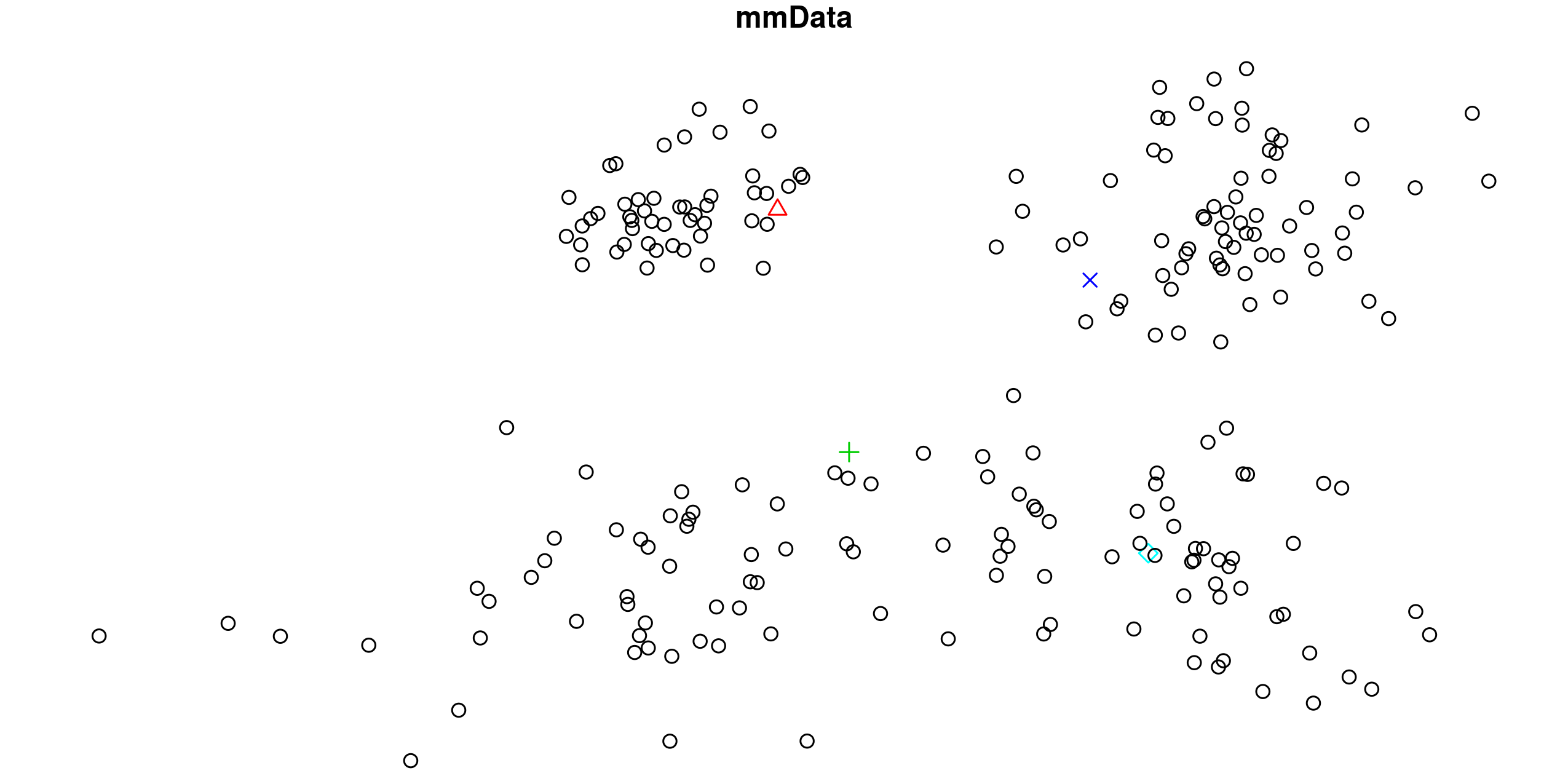With DP-MM, one only need to 1. update the DP prior (as defined in previous R example) with the information of the 4 observed samples, and 2. use the updated prior as the prior of the Gibbs sampling procedure. These 2 steps can be achieved by adding following code after obj <- DP(...) in the previous R example: ## 1. add the information of the 4 observed samples to the DP object ssObserved <- sufficientStatistics(obj=obj,x=mmData[c(50,100,150,200),,drop=FALSE],foreach = TRUE) for(i in 1L:4L) posterior(obj = obj,ss = ssObserved[[i]], z = i) # the choice of cluster label 'z' for the 4 observed samples are arbitrary, as long as they are different from each other. In this example I simply use z=1L:4L. ## 2. remove the 4 samples from the upcoming Gibbs sampling procedure mmData <- mmData[-c(50,100,150,200),] Run the code, and the result will be: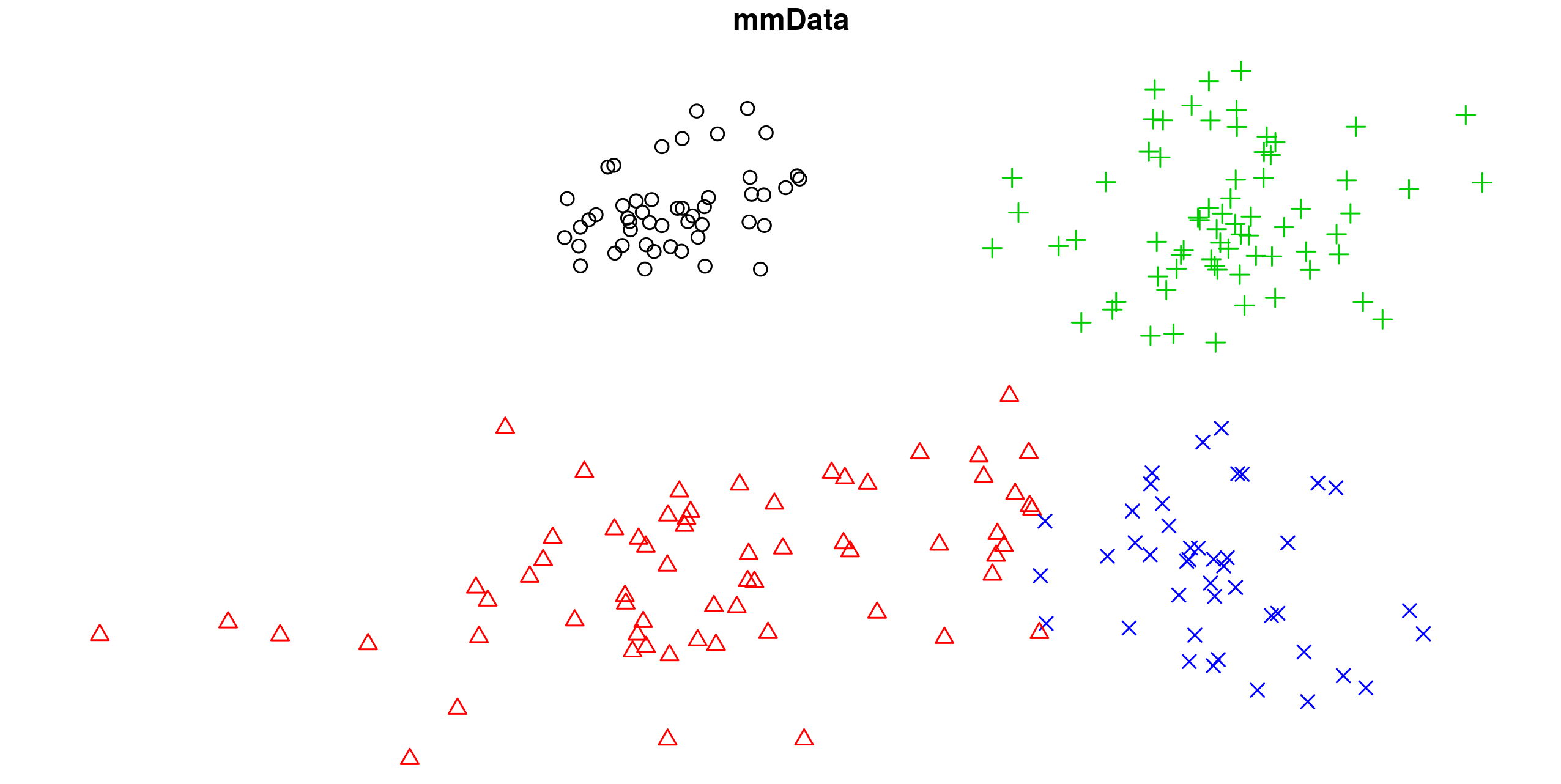### Hierarchical Mixture Models In a hierarchical mixture model, the observation $$x$$ are generated by some unknown mixture components and are split into $$J$$ groups, all $$J$$ groups share the same set of mixture components but with different mixture weights. Hierarchical Dirichlet Process(HDP) is a natural representation of a hierarchical mixture model, It has following graph structure: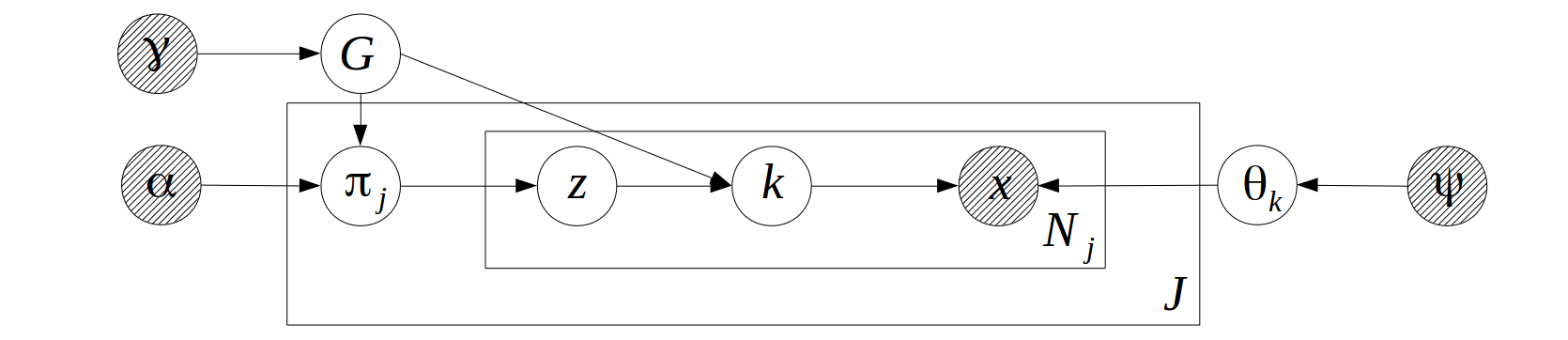If the component distribution is Gaussian, the CPDs will be: \begin{align} G|\gamma & \sim DP(\gamma) \\ \pi_j |\alpha,G & \sim DP(\alpha,G) \\ z | \pi_j & \sim Categorical(\pi_j) \\ k| z,G & \sim Categorical(G) \text{, if }z\text{ is a sample from } G\\ \theta_k | \psi & \sim NIW(\psi) \\ x|\theta_k & \sim Gaussian(\theta_k) \end{align} Where $$DP(\gamma)$$ is a Dirichlet process on positive integers with “concentration parameter” $$\gamma$$, the “base measure”, which is an uniform distribution on positive integers, is omitted from the formula. $$DP(\alpha,G)$$ is a Dirichlet process with concentration parameter $$\alpha$$ and base measure $$G$$. $$NIW(\psi)$$ is the Normal-Inverse-Wishart distribution with parameter $$\psi = (m,k,v,S)$$. $$m$$ is a numeric vector representing the “location parameter”, $$S$$ is a symmetric positive definitive matrix representing the “scale parameter”, $$k$$ and $$v$$ are degree of freedoms.The distribution of $$(\gamma, \alpha, G, \pi_j , z, k)$$ is a “HDP on positive integers”. HDP are usually represented in a much simpler and compact way(though not easier to use in practice, especially when generating random samples from HDP) in most literature: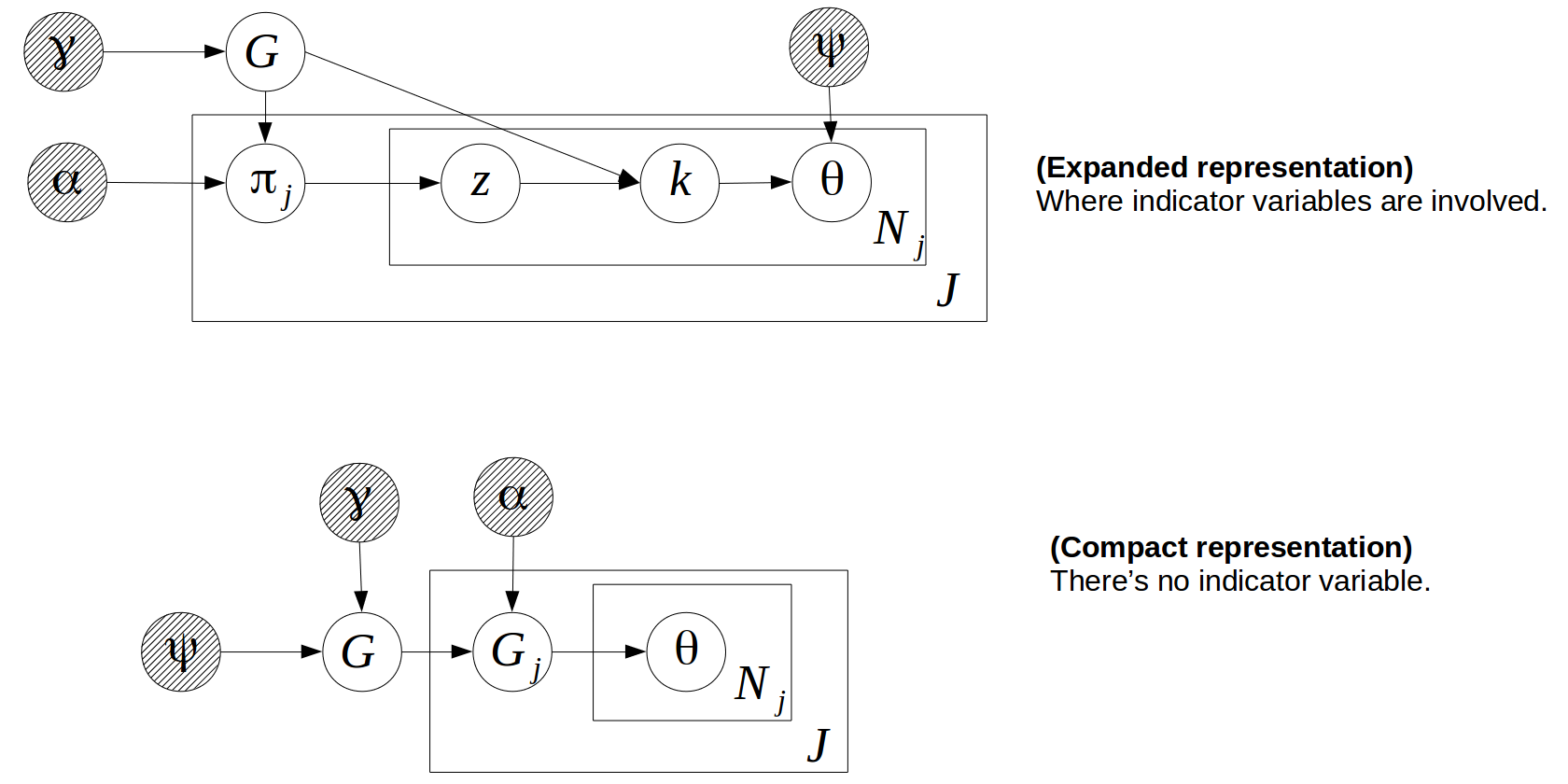From the compact representation we can see that HDP is following the “Hierarchical Bayesian” structure shown in Mindset graph $$(c)$$. In bbricks, “HDP on positive integers” is represented by an object of type "CatHDP". To further simplify the mixture model calculations, bbricks alos provides an "HDP" type to represent more general hierarchical Dirichlet process models. An object of type "HDP" is in essence a combination of a "CatHDP" object, which encodes the distribution of $$(\gamma, \alpha, G, \pi_j , z, k)$$, i.e. a HDP on positive integers; and an arbitrary "BasicBayesian" object, which encodes the $$\psi \rightarrow \theta_k \rightarrow x$$ structure. (in bbricks, all models with same structure as Mindset graph $$(b)$$ are "BasicBayesian"s, such as "GaussianNIW", "GaussianNIG" ,"CatDirichlet" and even "CatDP") To estimate $$k$$, we use the following Gibbs sampling procedure: 1. sample $$z_i,k_i$$ from $$z_i,k_i|z_{-i},k_{-i},\alpha,x,\gamma$$. R code: ## Learn HDP-MM posteriors using Gibbs sampling library(bbricks) ## load some mixture of Gaussian samples. ## mmhData is a list of two elements. mmhDatax is a matrix of Gaussian observations, each row is an observation; mmhData$groupLabel is the group label of each observation. ## see ?mmhData for details data(mmhData) x <- mmhData$x
js <- mmhData$groupLabel ## Step1: Initialization------------------------------------------ maxit <- 50 #iterative for maxit times burnin <- 30 #number of burn in samples ## create a HDP object to track all the changes, the HDP object in this case is a combination of a CatHDP object and a GaussianNIW object: obj <- HDP(gamma = list(gamma=1,j=max(js),alpha=1, H0aF="GaussianNIW", parH0=list(m=c(0,0),k=0.001,v=2,S=diag(2)*0.01))) ss <- sufficientStatistics(obj$H,x=x,foreach = TRUE) #sufficient statistics
set.seed(1)
z <- rep(1L,nrow(x))
k <- matrix(1L,nrow(x),maxit-burnin)    #place-holder for the sampled k
N <- length(ss)
for(i in 1L:N){# initialize k and z
tmp <- rPosteriorPredictive(obj = obj,n=1,x=x[i,,drop=FALSE],j=js[i])
z[i] <- tmp["z"]
k[i,1] <- tmp["k"]
posterior.HDP(obj = obj,ss = ss[[i]],ss1 = k[i],ss2 = z[i],j = js[i])
}
## Step2: main Gibbs loop---------------------------------------------
it <- 1                                 #iteration tracker
pb <- txtProgressBar(min = 0,max = maxit,style = 3)
while(it<=maxit){
if(it>burnin) colIdx <- it-burnin
else colIdx <- 1
for(i in 1L:N){
## remove the sample from the posterior info
## resample a new partition
tmp <- rPosteriorPredictive(obj = obj,n=1,x=x[i,,drop=FALSE],j=js[i])
z[i] <- tmp["z"]
k[i,colIdx] <- tmp["k"]
## add the information of the new sample
posterior(obj = obj,ss = ss[[i]], ss1=k[i,colIdx],ss2 = z[i],j=js[i])
}
if(it>burnin & colIdx<ncol(k)) k[,colIdx+1] <- k[,colIdx] #copy result of previous iteration
it <- it+1
plot(x=x[,1],y=x[,2],col=k[,colIdx])         #to visualize the group label dynamics
setTxtProgressBar(pb,it)
}
## Step3: Estimate group labels of each observation---------------
## pick the most frequent k as the best estimate
kBest <- apply(k,1,function(l){
tmp <- table(l)
names(tmp)[which.max(tmp)]
})
plot(x=x[,1],y=x[,2],col=kBest)

#### Topic Modeling with HDP

A topic model is a hierarchical mixture model(See Hierarchical Mixture Models) with categorical component distribution: \begin{align} G|\gamma & \sim DP(\gamma) \\ \pi_j |\alpha,G & \sim DP(\alpha,G) \\ z | \pi_j & \sim Categorical(\pi_j) \\ k| z,G & \sim Categorical(G) \text{, if }z\text{ is a sample from } G\\ \theta_k | \psi & \sim Dirichlet(\psi) \\ x|\theta_k & \sim Categorical(\theta_k) \end{align} The Gibbs sampling procedure on this model is exactly the same as the one in Hierarchical Mixture Models

R code:

## Learn HDP-LDA posteriors of the farm-ads corpus

word <- farmadsData$word document <- farmadsData$document
## Step1: Initialization------------------------------------------
set.seed(1)
maxit <- 30                            #iterative for maxit times
z <- rep(1L,length(word))
k <- rep(1L,length(word))
## initialize
uniqueWords <- unique(word)
obj <- HDP(gamma = list(gamma=1,j=max(document),alpha=1,H0aF="CatDirichlet",parH0=list(alpha=rep(0.5,length(uniqueWords)),uniqueLabels=uniqueWords))) #create a HDP object to track all the changes, the HDP object in this case is a combination of a CatHDP object and a CatDrirchlet object
N <- length(word)
## initialize k and z
for(i in 1L:N){
tmp <- rPosteriorPredictive(obj = obj,n=1,x=word[i],j=document[i])
z[i] <- tmp["z"]
k[i] <- tmp["k"]
posterior(obj = obj,ss = word[i], ss2 = z[i],j=document[i],ss1=k[i])
}
## Step2: main Gibbs loop---------------------------------------------
it <- 1                                 #iteration tracker
pb <- txtProgressBar(min = 0,max = maxit,style = 3)
while(it<=maxit){
for(i in 1L:N){
posteriorDiscard.HDP(obj = obj,ss = word[i],ss1=k[i],ss2=z[i],j=document[i]) #remove the sample information from the posterior
tmp <- rPosteriorPredictive(obj = obj,n=1,x=word[i],j=document[i])   #get a new sample
z[i] <- tmp["z"]
k[i] <- tmp["k"]
posterior(obj = obj,ss = word[i],ss1=k[i], ss2 = z[i],j=document[i]) #add the new sample information to the posterior
}
it <- it+1
setTxtProgressBar(pb,it)
}
## Step3: plot the result --------------------------------------------
## see which topics are most frequently appeared:
order(sapply(obj$X,function(l){sum(l$gamma$alpha)}),decreasing = TRUE) ## seems topic 2 and 1 appear the most, let's plot them: ## install.packages("wordcloud") # for word-cloud ## install.packages("RColorBrewer") # color palettes ## print topic 1 wordcloud:: wordcloud(words = obj$X[]$gamma$uniqueLabels,
freq = obj$X[]$gamma$alpha, min.freq = 1, max.words=100, random.order=FALSE, rot.per=0.35, colors=RColorBrewer::brewer.pal(5, "Set1")) ## print topic 2 wordcloud:: wordcloud(words = obj$X[]$gamma$uniqueLabels,
freq = obj$X[]$gamma\$alpha,
min.freq = 1,
max.words=100,
random.order=FALSE, rot.per=0.35,
colors=RColorBrewer::brewer.pal(5, "Set1"))

### Hierarchical Mixture Models with Two Layers of Hierarchies

By extending the Hierarchical Mixture Models with an additional layer of Dirichlet process on top, the model is then able to capture longer-term and cross-group dependencies. In this hierarchical mixture model, the observation $$x$$ are generated by some unknown mixture components and are split into $$M$$ groups, each group has $$J_m,m=1,...,M$$ sub-groups. All $$M$$ groups share the same set of mixture components but with different mixture weights, all $$J_m$$ sub-groups within each group $$m$$ also share the same set of mixture components but different mixture weights. The graphical model structure is: